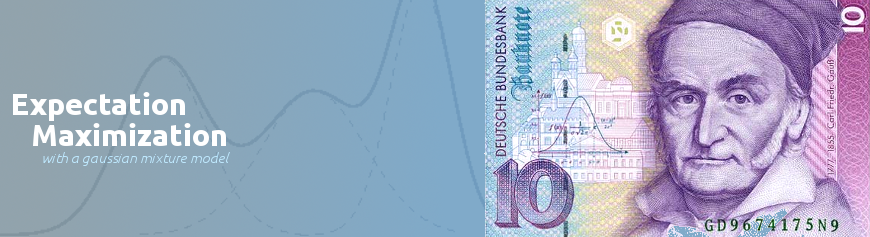## Expectation Maximization with Gaussian Mixture Models

Learn how to model multivariate data with a Gaussian Mixture Model. For training this model, we use a technique called Expectation Maximization.

## Introduction

Series: Expectation Maximization with Gaussian Mixture Models:

1. Introduction
2. One dimensional mixture

Gaussian Mixture Models are one of those basic machine learning techniques that every ML enthusiast should have in their toolbag. It lets you model a large class of datasets and work with them efficiently. The probability theory behind this tool may seem hard to grasp - but I'll try and teach you how these things actually work.

## The plan

The goal of this series is to teach you about expectation maximization using Gaussian Mixture Models. Expectation Maximization (EM) is a general technique and is not related to GMMs at all. You can apply EM to GMMs, Markov random fields and pretty much any situation where you need to guess some model parameters.

In this series, I will first introduce you to learning a simple 1D Gaussian model (not a mixture). We will then extend it to a mixture of 1D gaussians (a linear combination of several gaussians). We will finally extend it to the most general form - multidimensional mixture of gaussians.

## One dimensional gaussian models

These are the simplest form. You have some 1D data and want to figure out what gaussian curve is the best. Our goal in this part is to learn a one dimensional gaussian model something like this: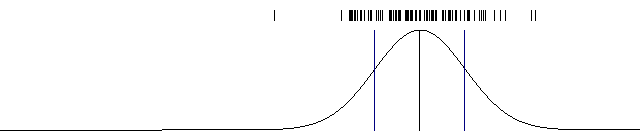A single one dimensional gaussian model. The data is shown at the top and the model at the bottom.

The Gaussian curve is defined using two numbers: the mean and the standard deviation. If we can somehow calculate these numbers for our data, we'll be able to gaussian model. These two parameters (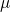and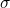) are needed per dimension - so if you have 1D data, you need one pair. If you have 3D data, you need six numbers and so on.

"Learning" a model is the same as "estimating" parameter value in statistics. This can help you form a bridge between machine learning and statistics.

The Gaussian function looks like this: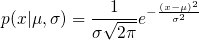If you know the meanand standard deviationof a model, you can predict the probability of a given data point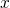. But we must first calculate these two parameters.

## Learning the one dimensional Gaussian

Learning a 1D Gaussian is very straightforward. In fact, it is taught in high school. To calculate the meanall you need to do is: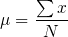You simply sum up all the data points and divide it by the number of data points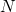.

Calculating the standard deviation is simple as well: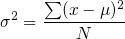A more computationally efficient method is to subtract the mean later on. This translates to fewer computations inside the `for` loop and is thus more efficient.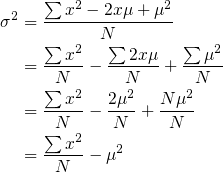## Implementing the learner

With these basics, we're now ready to learn these gaussian models. I'll be using OpenCV (because it comes with basic linear algebra tools) and the C++ STL for generating random numbers.

We're not doing expectation maximization just yet. A single gaussian has no need for such a technique. When we get to a mixture of gaussian models, I'll introduce EM to you.

Let's start out with the basics.

```#include <stdio.h>
#include <opencv2/opencv.hpp>
#include <random>

int main(int argc, char* argv[]) {
run_gaussian_1d();
return 0;
}
```

This is the standard template for starding out with an OpenCV project. I've created a function called `run_gaussian_1d()` - we'll be creating more functions over the next few parts of this tutorial.

Let's define this function now.

```void run_gaussian_1d() {
const int count = 1000;
const float mean = (rand()/(float)RAND_MAX-0.5f) * 320 + 320;
const float std  = (rand()/(float)RAND_MAX) * 100;
```

We randomly pick a mean and standard deviation. The value of `mean` centered somewhere between 160 and 480. The value of `std` is just any number from 0 to 100.

```float* data = generate_1d_data(mean, std, count);
```

Then we generate our training data. The data will follow the provided mean and std value and will return `count` data points. Since we're dealing with just 1D things at the moment, the data will simply be an array of floats.

```float learned_mean=0, learned_Variance=0;
for(int i=0;i<count;i++) {
learned_mean += data[i];
learned_variance += data[i]*data[i];
}

learned_mean /= count;
learned_variance = learned_variance/count - learned_mean*learned_mean;
float learned_std = sqrt(learned_variance);
```

We use the formulae mentioned above to "learn" the parameters. This is very straightforward. Finally, let's generate a visual image to see what was learned.

```    const int graph_width = 640;
const int graph_height = 140;
cv::Mat graph(graph_height, graph_width, CV_8UC3, cv::Scalar(255, 255, 255));
draw_1d_data(graph, data, count);
draw_1d_gaussian(graph, learned_mean, learned_std);

printf("Original mean = %0.2f, std = %0.2f\n", mean, std);
printf("Learned mean = %0.2f, std = %0.2f\n", learned_mean, learned_std);
cv::imwrite("./1d-single-gaussian.png", graph);

delete[] data;
}
```

We've not written most of the functions here - we'll get to them in a bit. We also print out the original mean/std used to generate the data and the mean/std learned by the model. Before that, we must write the function to generate our data.

```float* generate_1d_data(float mean, float std, int count) {
float* data = new float[count];
```

We start out by allocating enough memory to hold all the numbers.

```    for(int i=0;i<count;i++) {
float noise = 2*std * (float)rand()/RAND_MAX;
noise -= 10;
data[i] = get_gaussian_random(mean, std) + noise;
}

return data;
}
```

We generate a random number as noise and add it to the pure gaussian generated by the function `get_gaussian_random`.

```float get_gaussian_random(float mean, float std) {
std::random_device rd;
std::mt19937 e2(rd());
std::normal_distribution<float> gen(mean, std);

return gen(e2);
}
```

Here's a function that uses the C++ STL to generate gaussian numbers with the provided `mean` and `std` standard deviation. Now we can draw some graphs.

## Drawing graphs

Let's start out by drawing the data points we've generated.

```void draw_1d_data(cv::Mat img, float* data, int count) {
for(int i=0;i<count;i++) {
float pt = data[i];
cv::line(img, cv::Point(pt, 10), cv::Point(pt, 20), cv::Scalar(0));
}
}
```

This is a simple function. It represents each data point as a short line.

```void draw_1d_gaussian(cv::Mat img, float mean, float stddev, cv::Scalar color=cv::Scalar(0, 0, 0)) {
float* prob = new float[img.cols];
float frac =1/(stddev * sqrt(2*M_PI));

float min_prob = FLT_MAX;
float max_prob = FLT_MIN;
```

We start out by allocating some points. The plan is, we'll evaluate the probability in the range zero to `img.cols` (which is 640 in our case). Since our mean is (conveniently) between 160 and 480, calculating probabilities between 0-640 should be sufficient.

```    for(int x=0;x<img.cols;x++) {
prob[x] = frac * exp( -0.5 * (x-mean)*(x-mean) / (stddev*stddev));

if(prob[x] > max_prob)
max_prob = prob[x];

if(prob[x] < min_prob)
min_prob = prob[x];
}
```

Here's we're actually calculating the probabilities and storing them into `prob`. We're doing this for the entire range and calculating the maximum and minimum values (so we can scale our graph appropriately).

```    float prev = 0;
for(int x=0;x<img.cols;x++) {
float p = 30 + 100*(1-(prob[x]/(max_prob-min_prob)));

cv::line(img, cv::Point(x-1, prev), cv::Point(x, p), color);
prev = p;
}
```

Here we do the actual drawing. This code ensures the graph starts at y=30 in the image, is 100 pixels in height and makes sure the 100 pixels cover the range `min_prob` to `max_prob`. Also, since the y coordinate axis is flipped from our usual convention (the y axis increase from top to bottom - but it increase from bottom to top in most diagrams). This flip is done by subtracting the correct answer from 1 (`1 - ...`).

```    cv::line(img, cv::Point(mean, 30) cv::Point(mean, 130), cv::Scalar(0));
cv::line(img, cv::Point(mean-stddev, 30), cv::Point(mean-stddev, 130), cv::Scalar(128));
cv::line(img, cv::Point(mean+stddev, 30), cv::Point(mean+stddev, 130), cv::Scalar(128));
}
```

We finish off the function by drawing the mean and one standard deviation to the left and one standard deviation to the right.Learning one single-dimensional gaussian in really easy.

## Wrap up

Well this was easy, right? We'll get into some juicy expectation maximization in the next part. We'll try and learn a mixture of gaussians in one dimension and then later on extend it to multiple dimensions. I hope you found this tutorial useful!

## More in the series

This tutorial is part of a series called Expectation Maximization with Gaussian Mixture Models:

1. Introduction
2. One dimensional mixture

Utkarsh Sinha created AI Shack in 2010 and has since been working on computer vision and related fields. He is currently at Microsoft working on computer vision.